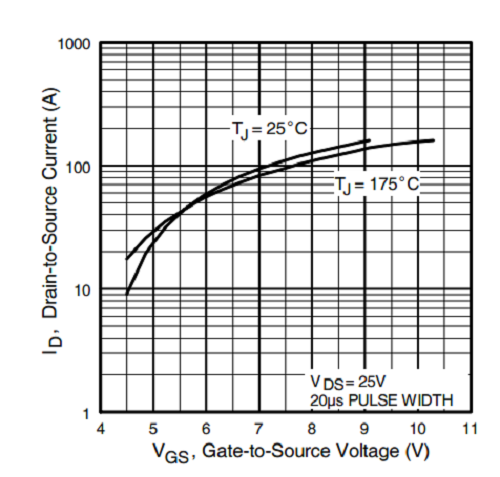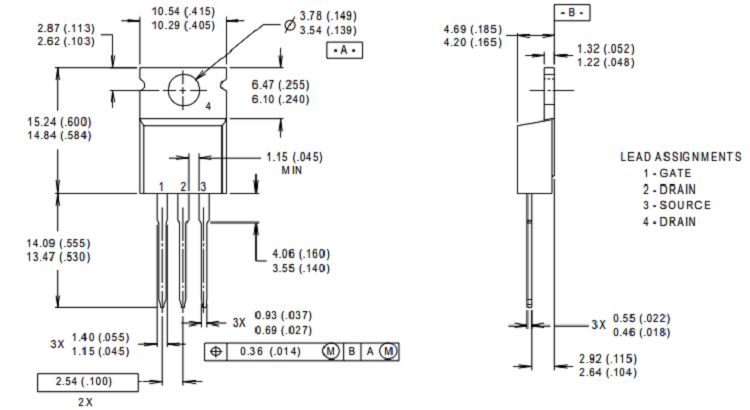# IRFZ44N N-Channel Power MOSFET

The IRFZ44N is a N-channel MOSFET with a high drain current of 49A and low Rds value of 17.5 mΩ. It also has a low threshold voltage of 4V at which the MOSFET will start conducting. Hence it is commonly used with microcontrollers to drive with 5V. However a driver circuit is needed if the MOSFET has to be switched in completely.

### IRFZ44N Pinout Configuration

 Pin Number Pin Name Description 1 Gate Controls the biasing of the MOSFET 2 Drain Current flows in through Drain 3 Source Current flows out through Source

### Features and Specifications

• Small signal N-Channel MOSFET
• Continuous Drain Current (ID) is 49A at 25°C
• Pulsed Drain Current (ID-peak) is 160A
• Minimum Gate threshold voltage (VGS-th) is 2V
• Maximum Gate threshold voltage (VGS-th) is 4V
• Gate-Source Voltage is (VGS) is ±20V (max)
• Maximum Drain-Source Voltage (VDS) is 55V
• Rise time and fall time is about 60ns and 45ns respectively.
• It is commonly used with Arduino, due to its low threshold current.
• Available in To-220 package

Note: Complete Technical Details can be found in the IRFZ44N datasheet given at the end of this page.

### Alternatives for IRFZ44N

IRF2807, IRFB3207, IRFB4710

### Where to use IRFZ44N MOSFET

The IRFZ44N is known for its high drain current and fast switching speed. Adding to that it also has a low Rds value which will help in increasing the efficiency of switching circuits. The MOSFET will start turning on with a small gate voltage of 4V, but the drain current will be maximum only when a gate voltage of 10V is applied. If the mosfet has to be driven directly from a microcontroller like Arduino then try the logic level version IRLZ44N mosfet.

### Difference between IRLZ44N and IRFZ44N Mosfet

The IRLZ44N and IRFZ44N MOSFETs are often confused among each other and used incorrectly. The IRLZ44N is a Logic level Mosfet with a very low gate threshold voltage of 5V, meaning the MOSFET can be fully turned on with just 5V on its gate pin which avoids the need for a driver circuit.

The IRFZ44N on the other hand requires a gate driver circuit if the MOSFET has to be turned on completely using a microcontroller like Arduino. However it does turn on partially with direct 5V form a I/O pin, but the output drain current will be limited.

### How to use IRFZ44N MOSFET

Unlike transistors MOSFET’s are voltage controlled devices. Meaning, they can be turned on or turned off by supplying the required Gate threshold voltage (VGS). IRFZ44N is an N-channel MOSFET, so the Drain and Source pins will be left open when there is no voltage applied to the gate pin. When a gate voltage is applied these pins gets closed.

If it is required to be switched with Arduino, then a simple drive circuit using a transistor will work to provide the required gate voltage to trigger the MOSFET to open fully. For other switching and amplifying applications, a dedicated MOFET Driver IC is required.

### IRFZ44N with 5V gate (Arduino)

If the MOSFET gate pin is directly connected to an I/O pin of a microcontroller like Arduino, PIC, etc. Then it will not open completely and the maximum drain current will depend on the voltage applied to the gate pin. The below graph shows how much drain current is permitted for gate threshold voltage from 4V to 10V.As you can see the MOSFET opens completely only when the gate voltage is around 10V. If it is somewhere around 5V then the drain current is limited to 20A and so on.

### Applications

• Switching high power devices
• Control speed of motors
• LED dimmers or flashers
• High Speed switching applications
• Converters or Inverter circuits

### 2D Model and Dimensions

If you are designing a PCB or Perf board with this component then the following picture from the Datasheet will be useful to know its package type and dimensions.Component Datasheet